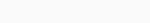# Physics

 1. Glass is also called ________.1. Supercooled liquid 2. Super liquid 3. Ideal liquid 4. Distilled liquidOption “1” is correct. Glass is sometimes called a super cooled liquid because it does not form a crystalline structure, but instead forms an amorphous solid that allows molecules in the material to continue to move. 2. An object at rest will remain at rest and an object in motion will remain in motion until and unless it is acted upon by an external force. This is Newton’s1. First law 2. Second law 3. Third law 4. Fourth lawOption “1” is correct. Newton’s First Law of Motion states that a body at rest will remain at rest unless an outside force acts on it, and a body in motion at a constant velocity will remain in motion in a straight line unless acted upon by an outside force. 3. What will happen if an object is dropped from a height and there is no air resistance? 1. It will fall with a constant speed and acceleration 2. Its acceleration will increase 3. Both speed and acceleration will increase 4. Its speed will increaseOption “4” is correct. When an Object is dropped from height and when there is no air resistance the speed of object will increase. 4. Steam locomotive was invented by?1. Simon Stevin 2. George Stephenson 3. Gary Starkweather 4. Percy SpencerOption “2” is correct. George Stephenson built the first steam locomotive to carry passengers on a public rail line, which is between Stockton and Darlington Railway in 1825. 5. If a body slides over a surface, the force resisting the motion between them is called ______. 1. Centripetal force 2. Friction 3. Centrifugal force 4. InertiaOption “2” is correct. Friction is the force resisting the relative motion of solid surfaces, fluid layers, and material elements sliding against each other. 6. In a streamline flow, ________ at every point in the fluid remains same.1. Force 2. Pressure 3. Velocity 4. SpeedOption “3” is correct. In a streamline flow, velocity at every point in the fluid remains same. 7. The value of acceleration due to gravity (g) at a distance of 2R from the surface of earth, where R is the radius of earth is ______.1. g/3 2. g/4 3. g/9 4. g/2 Option “3” is correct.8. Meter in a vehicle that calculates distance covered by the vehicle is called __________.1. Speedometer 2. Odometer 3. Thermometer 4. TDS meterOption “2” is correct. An odometer or odograph is an instrument for measuring the distance travelled by a vehicle, such as a bicycle or car. The device may be electronic, mechanical, or a combination of the two. 9. What is the SI unit of pressure?1. Newton 2. Weber 3. Pascal 4. HenryOption “3” is correct. The SI unit for pressure is the pascal (Pa), equal to one newton per square metreThis name for the unit was added in 1971. It is named after the French polymath Blaise Pascal. 10. The time taken by a pendulum to complete one oscillation is called its? 1. Maximum speed 2. Average speed 3. Time period 4. Time intervalOption “3” is correct. The time taken by a pendulum to complete one oscillation is called its Time Period.